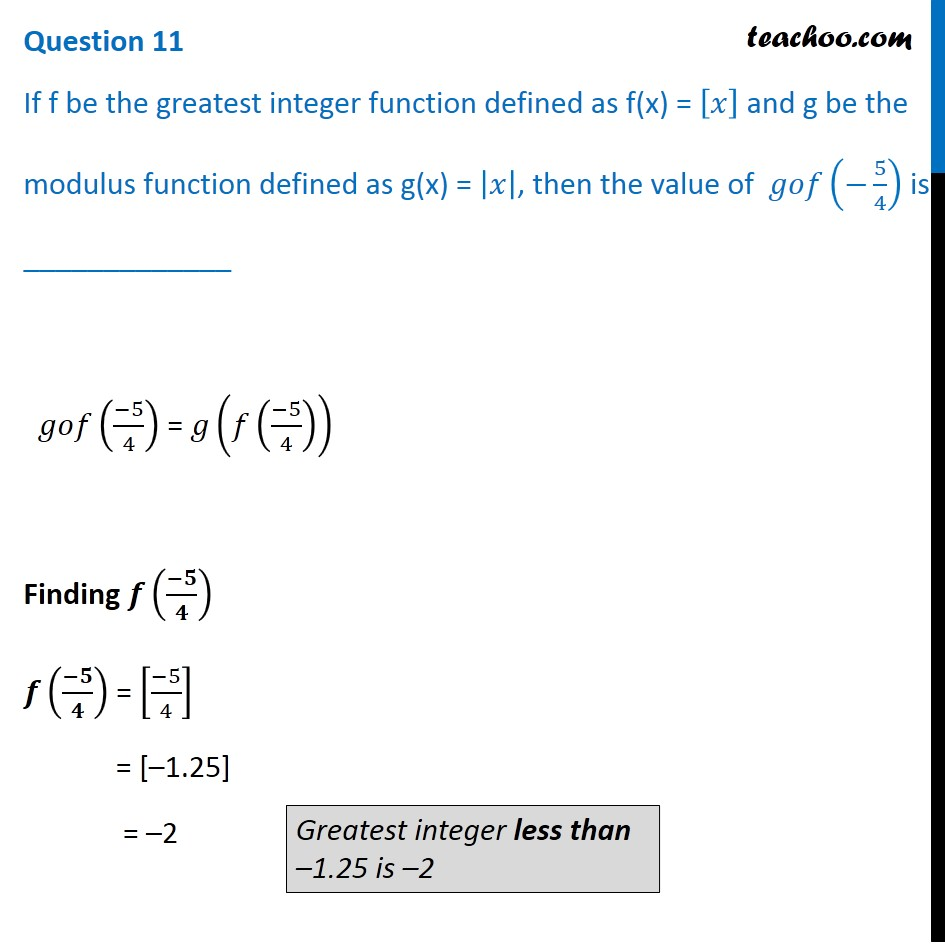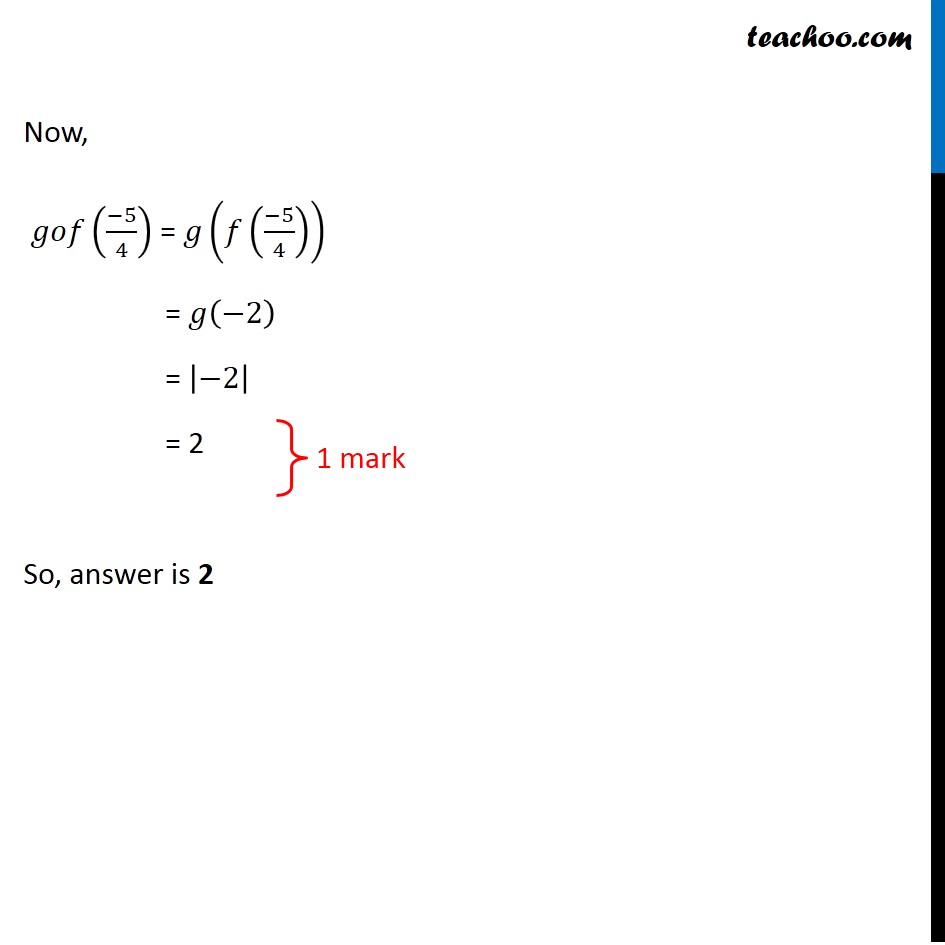CBSE Class 12 Sample Paper for 2020 Boards

Class 12
Solutions of Sample Papers and Past Year Papers - for Class 12 Boards

## If f be the greatest integer function defined as f(x) = [x] and g be the modulus function defined as g(x) = |x|, then the value of  gof(-5/4) is _____________### Transcript

Question 11 If f be the greatest integer function defined as f(x) = [𝑥] and g be the modulus function defined as g(x) = |𝑥|, then the value of 𝑔𝑜𝑓(−5/4) is _____________ 𝑔𝑜𝑓((−5)/4) = 𝑔(𝑓((−5)/4)) Finding 𝒇((−𝟓)/𝟒) 𝒇((−𝟓)/𝟒) = [(−5)/4] = [–1.25] = –2 Greatest integer less than –1.25 is –2 Now, 𝑔𝑜𝑓((−5)/4) = 𝑔(𝑓((−5)/4)) = 𝑔(−2) = |−2| = 2 So, answer is 2